## Find the resultant R of the following forces all acting on the same point in the given directions: 30lb northeast; 70 lb to the south; and 5

Question

Find the resultant R of the following forces all acting on the same point in the given directions: 30lb northeast; 70 lb to the south; and 50 lb 20 degrees north of west.

in progress 0
3 weeks 2021-08-23T23:09:26+00:00 1 Answers 2 views 0

net force is 40.8 Lb in direction 50.8 degree South of West

Explanation:

As we know that the three forces are given as

F1 = 30 lb North East

F2 = 70 lb South

F3 = 50 lb at 20 degree North of West

Now we can write in vector form as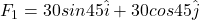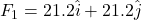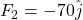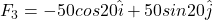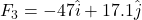now the net force due to above all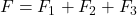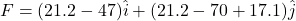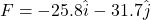magnitude of net resultant force is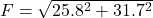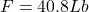direction of the force is along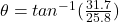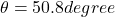So net force is 40.8 Lb in direction 50.8 degree South of West